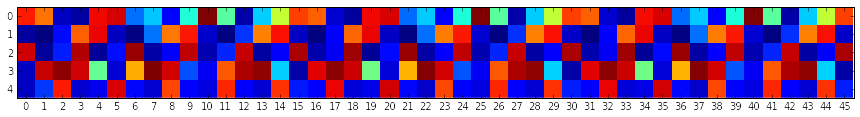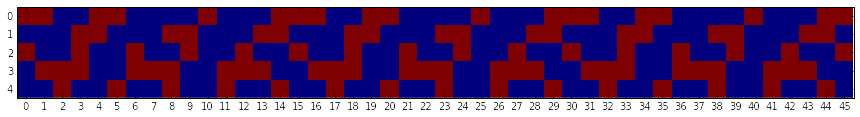## FizzBuzz in Theano, or, Backpropaganda Through Time.

Interviewer: Hi! Can I get you coffee or anything? Do you need a break?

Me: No, but thanks!

Interviewer: Let’s get started then! Are you familiar with “fizzbuzz”?

Me: … I know about 15 fizzbuzz different ways to do it. See what I did there?

Interviewer: Right, so I need you to print the numbers from 1 to 100, except that if the number is divisible-

Me: I said I know the problem. I’ll code in python.

Interviewer: That’s fine.

Me: Some standard imports…

In [ ]:
import theano_toolkit
import theano
import theano.tensor as T
import numpy as np

from theano_toolkit.parameters import Parameters
from theano_toolkit import updates


Interviewer: Geez another neural nut…

Me: What?

Interviewer: I said that’s a good start. Go on.

Me: We’re gonna have to generate labels to train on, so let’s implement fizzbuzz…

In :
def fizzbuzz(N):
# 0 for copy,
# 1 for fizz,
# 2 for buzz,
# 3 for fizzbuzz
output = np.zeros((N,),dtype=np.int8)
idx = np.arange(N)
output[(idx%3)==0] = 1
output[(idx%5)==0] = 2
output[(idx%15)==0] = 3
return output


Interviewer: Okay, that’s enough, that function already demonstrates you know the problem, and frankly, sitting through this once is enough.

Me: Aha! But I’m betting the other candidate didn’t use RNNs did he?

Interviewer: Wha-

Me: Recurrent neural networks! It can solve all problems known to man! Let’s initialise some model parameters.

In :
hidden_size = 5
P = Parameters()
P.W_hidden_hidden = 0.1 * np.random.randn(hidden_size,hidden_size)
P.b_hidden = np.zeros(hidden_size)
P.init_hidden = 0.1 * np.random.randn(hidden_size)
P.W_output = np.zeros((hidden_size,4))
P.b_output = np.zeros(4)
parameters = P.values()


Interviewer: Only 5 hidden units?

Me: Oh, that’s a good question I-

Interviewer: You know what? Pretend I never asked.

Me: It’s okay I’ll explain later, but success is guaranteed. Let’s define the model…

In :
x = T.iscalar("x")
lbls = T.ivector("lbls")
def step(prev_hidden):
return T.nnet.sigmoid(T.dot(prev_hidden,P.W_hidden_hidden) + P.b_hidden)

init = T.nnet.sigmoid(P.init_hidden)
hiddens,_ = theano.scan(
fn=step,
outputs_info=[init],
n_steps=x
)
outputs = T.nnet.softmax(T.dot(hiddens,P.W_output) + P.b_output)


Me: We need to do that concatenation of the first hidden representation or we’d have an off by one error.

Interviewer: Yawns

Me: Okay let’s train this using the standard cross-entropy cost, with an L2 penalty…

In :
loss = T.mean(T.nnet.categorical_crossentropy(outputs,lbls))
cost = loss + np.float32(1e-4) * sum(T.sum(T.sqr(w)) for w in parameters)


Me: Great, now we’ll prepare three functions for training, validation, and a third one just for analysis purposes later…

Interviewer: Zzzzz…

In :
train_fizzbuzz = theano.function(inputs=[x,lbls],outputs=cost,
fizzbuzz_accuracy = theano.function(inputs=[x,lbls],
outputs=[
T.mean(T.neq(T.argmax(outputs,axis=1),lbls)),
loss
])
fizzbuzz_hiddens = theano.function(inputs=[x],outputs=hiddens)


Me: Time to train this thing! So, I’m thinking… 200,000 iterations, with a checkpoint every 10,000 iterations. We’ll validate it on a longer fizzbuzz sequence to make sure it’s generalising right.

Inteviewer: Chokes while snoring

In :
min_val = np.inf
model = []
for _ in xrange(20):
for _ in xrange(10000):
length = np.random.randint(4) * 15 + 16
train_fizzbuzz(length,fizzbuzz(length))
acc, val_cost = fizzbuzz_accuracy(301,fizzbuzz(301))
print acc, val_cost,
if val_cost < min_val:
model = [ w.get_value() for w in parameters ]
min_val = val_cost
print "Save"
else:
print

0.332225913621 0.764216542244 Save
0.332225913621 0.662550151348 Save
0.325581395349 0.651876211166 Save
0.325581395349 0.648342370987 Save
0.325581395349 0.649337947369
0.318936877076 0.631394803524 Save
0.0 0.0484583750367 Save
0.0 0.0167257916182 Save
0.0 0.0113316513598 Save
0.0 0.0111983753741 Save
0.0 0.0111334351823 Save
0.0 0.0111326910555 Save
0.0 0.0111096147448 Save
0.0 0.0111302910373
0.0 0.0111406939104
0.0 0.0111133335158
0.0 0.0110623119399 Save
0.0 0.0111135747284
0.0 0.0111036580056
0.0 0.0110982181504


Me: And we’re done-

Interviewer: Wakes up Wh- Oh. Great! Finally.

Me: -with the training. We have to analyse the model’s hidden states now..

Interviewer: Uh. That’s fine. We’ll get back to you, thanks for coming out to interview today, bye! Sneaks out the door

Me: I’ll just load the best model based on the “validation” we have and test it.

In :
for w,w_save in zip(parameters,model): w.set_value(w_save)
test_length = 46
hidden_states = fizzbuzz_hiddens(46).T

In :
import matplotlib.pyplot as plt
import matplotlib
%matplotlib inline
plt.figure(figsize=(15,5))
plt.xticks(np.arange(test_length))
plt.imshow(hidden_states,interpolation='None')

Out:
<matplotlib.image.AxesImage at 0x7fa9ca2b7910>Me: Let me just clean that up..

In :
plt.figure(figsize=(15,5))
plt.xticks(np.arange(test_length))
plt.imshow(hidden_states>0.5,interpolation='None')

Out:
<matplotlib.image.AxesImage at 0x7fa9ca18fdd0>Me: So this diagram shows the hidden state at every iteration, it’s a bit how our own neurons, and this is how the brain works. Check this out. There’s a- Wait, where’s everyone?

I was thinking about Joel Grus’ parody about learning fizz buzz using neural networks, and I felt like it’d be an fun way to demonstrate learning some kind of state machine using RNNs.

His approach was using a binary encoding of the input and making a prediction about fizzing or buzzing. That actually seems like a harder problem to me, just thinking about it in terms of boolean operations that need to be performed. Moreover, it doesn’t generalise to numbers bigger than the one pre-defined. Another way of approaching this without that limitation would then be to count up from 1 and output as we go. Deterministic finite-state automatons (DFAs) do that very nicely, and it’s easy to see how a DFA could be encoded in an RNN. And even though the hidden states of an RNN are not finite, in practice, it is possible to learn a working DFA with an RNN. There’s been literature (that I haven’t read) on the relationship between them that date back to it’s inception.

I figured in order for this to happen, conceptually, there’d be 2 state machines running in “parallel”: A 3-state DFA maintaining divisibility by 3, and a 5-state DFA maintaining divisibility by 5. 3-states requires 2 bits to encode, 5-states requires 3, so together, 5 hidden units. We might even be able to bring that down to 4 hidden units, seeing as the product of these two DFAs would have 15 states, which would require 4 bits to encode.

If you look at that last diagram, there’s a repeating pattern from one “fizzbuzz” to another. The cleaned version shows a similar state between (6, 12), (21, 27), and (36, 42), but looking at the uncleaned version, you’ll see that the activation isn’t so strong in one of the units. There are other patterns just looking at individual rows of the hidden activations too. I was hoping there would be an obvious pattern where a unit would be activated every 3 iterations (there is: see row 2) and another activated every 5 (row 0 sort of does this, but not as consistently as I’d like), and both of these would be activated together every 15.

It would be great if the hidden layer size for real-life problems could be worked out in a similar way. Hopefully one day we can!